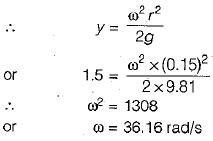Courses

# Test: Kinematics of Flow & Ideal Flow - 1

## 10 Questions MCQ Test Topicwise Question Bank for Mechanical Engineering | Test: Kinematics of Flow & Ideal Flow - 1

Description
This mock test of Test: Kinematics of Flow & Ideal Flow - 1 for Mechanical Engineering helps you for every Mechanical Engineering entrance exam. This contains 10 Multiple Choice Questions for Mechanical Engineering Test: Kinematics of Flow & Ideal Flow - 1 (mcq) to study with solutions a complete question bank. The solved questions answers in this Test: Kinematics of Flow & Ideal Flow - 1 quiz give you a good mix of easy questions and tough questions. Mechanical Engineering students definitely take this Test: Kinematics of Flow & Ideal Flow - 1 exercise for a better result in the exam. You can find other Test: Kinematics of Flow & Ideal Flow - 1 extra questions, long questions & short questions for Mechanical Engineering on EduRev as well by searching above.
QUESTION: 1

### For the velocity function,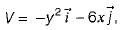the equation of the streamline passing through (1,1) is

Solution: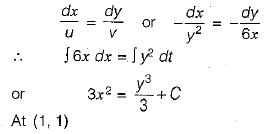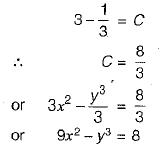QUESTION: 2

### A steady, incompressible, 2-D velocity field is given by u = x + y + 2 v = x - y - 3 The coordinate of stagnation point (if it exists) is

Solution: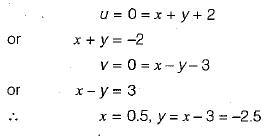QUESTION: 3

### The velocity along the centreline of a nozzle of length L is given by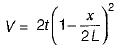where, V - velocity in m/s, t = time in seconds from commencement of flow, x = distance from inlet to the nozzle The total acceleration when t = 3s, x = 0.5 m and L - 1 m is

Solution: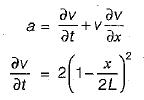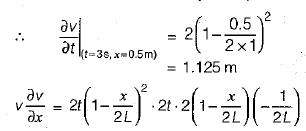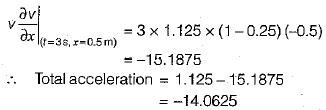QUESTION: 4

A pathline represents

Solution:
QUESTION: 5

There is no geometrical distinction between the streamline, pathline and streakline in case of

Solution:
QUESTION: 6

Which of the following statement is wrong?

Solution:

Streamline spacing varies inversely as the velocity; converging of streamline in any particular direction shows accelerated flow in that direction.

QUESTION: 7

When fluid flows in a curved path and requires no external impressed constant force, the fluid motion is known as

Solution:
QUESTION: 8

Match List-I (Example of flow) with List-Il (Type of flow) the following: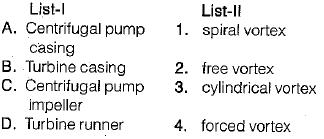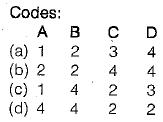Solution:
QUESTION: 9

When a cylindrical vessel containing a liquid is revolved at constant angular velocity about its vertical axis, the pressure

Solution: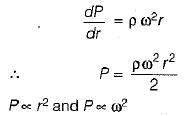QUESTION: 10

An open cylindrical tank is 15 cm (radius) x 150 cm (height) and is filled with water to a depth of 80 cm. What is the maximum angular velocity with which it can be rotated so that center of the bottom of the tank is just exposed?

Solution:

When the point at the center of the base is just exposed, the depth of fluid at center becomes zero. Therefore, the depth of parabola equals the height of tank, y = 150 cm